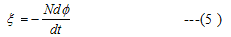meta name="description" content="Learn about Magnetic Flux, Faraday's Law and Lenz's Law "> Direction of Induced EMF: Lenz's Law|ElectroMagnetic induction# Lenz's Law

## Direction of Induced EMF: Lenz's Law

• The direction of induced current and emf is given by lenz's law
• Due to change in magnetic flux through a closed loop an induced current is established in the loop
• Lenz's law states that
'The induced current due to the induced emf always flow in such a direction as to oppose the change causing it'
• Now we can combine faraday's law as given in equation (4) to find the direction of emf
• Thus we can say that "The emf induced in a coil is equal to the negative rate of the change of the magnetic flux linked with it "## Explaination of lenz's law

• To explain this law again consider faradays experiment 1 in which north pole of the magnet moves towards a closed coil
• This movement of north pole of magnetic induces current in the coil in such a direction so that end of the coil ,facing and approaching north pole becomes a magnetic north pole
• The repulsion between two poles opposes the motion of the magnet towards the coil
• Thus work has to be done to push the magnet against the coil
• It is this mechanical work which causes the current to flow in the coil against its resistance R and supply the energy for the heat loss
• The mechanical workdone is converted to electrical energy which produces the heat energy
• If the direction of the induced current were such as not to oppsose the motion ,then we would be obtaining electrical energy continously without doing any work ,which is impossible
• So ,every things seems to be all right if we accept lenz's law otherwise the principle of conservation of the energy would be voilated
• Direction of the induced current can be found using Fleming right hand rule.
" If we stretch thumb ,index and middle finger perpendicular to one another then index fingers points in direction of the magnetic field ,middle finger in direction of induced current and thum points in direction of the motion of the conducter"